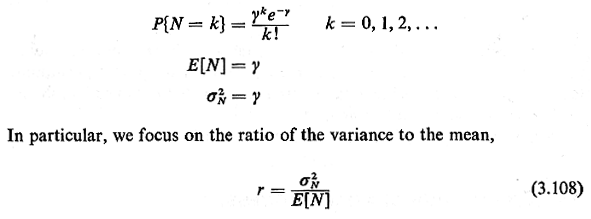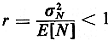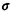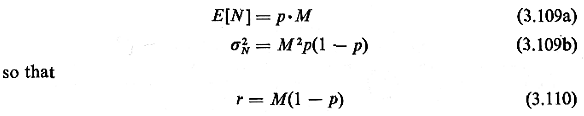## 3.9 ALTERNATIVE SPATIAL PROCESSES

 The spatial Poisson process has a "no memory" property similar to that of the time Poisson process. In this case, the existence or nonexistence of a Poisson entity in any region of space does not influence the likelihood of other Poisson entities existing in nearby disjoint regions. Moreover if we know that there are n points distributed in a fixed region of area A and that these points were generated from a spatial Poisson process, then the n points are independently uniformly distributed over the region. This is simply the twodimensional generalization of the "unordered arrival times" argument made in Chapter 2 for Poisson processes in time.     However, many naturally occurring processes do not adhere to the Poisson assumptions. For instance, one can imagine certain processes for which the existence of one point would increase the likelihood of other points occurring nearby. Such "clustering" processes could include hospitals (which cluster due to economies of scale), certain industries, police cars, crimes, households having certain demographic characteristics, and so on. For these processes the spatial Poisson process is an inadequate model. Similarly, one can imagine other processes for which the existence of one point would decrease the likelihood of other points occurring nearby. Such "spread" processes could include certain retail establishments (e.g., supermarkets, hamburger havens), urban service facilities (e.g., libraries, outpatient clinics, "little city halls"), and street intersections.     The question is, how do we model such processes? The answer, at present, is that models for such complicated spatially dependent processes are quite inadequate. While we can quite successfully generalize from Poisson processes to renewal processes in time, there does not seem to be an analogous generalization for spatial processes. Still, urban geographers have devised various techniques for tackling this problem, and here we illustrate a popular one based on partitioning the city into a regular lattice of equal size small cells.     Suppose that we are interested in the number of points N in a particular cell of unit area. If the points are distributed according to a spatial Poisson process with parameter Y (points/unit area), then `` For a spatial Poisson process, r = 1. Now, for a "spread" process in which the existence of one point reduces the chance of another point being located nearby, assuming that means are kept constant, one would expect that the Poisson distribution could be modified in a way that would take probabilities away from the tails of the Poisson distribution and add these to probabilities near the mean (center) of the distribution. Such a modification would have the effect of reducing the variance of the distribution. Thus, any spatial process for which `````` is called a spread process  (meaning points tend to be "spread out" over the Plane).  An extreme case occurs with a perfectly   regular lattice of points which provides each geographical cell with exactly the same number of points; then2N = 0, so that r = 0. A spread process, sometimes also called a regular process, because of its closeness to a regular lattice, includes the class of processes ranging from a perfect lattice of points to (but not including) the Poisson process. A clustered process, on the other hand, would probably have many cells with zero points and others with more than predicted by the Poisson model. Thus, again keeping means constant, one could obtain a pmf for a clustered process by taking probability away from the integer values near the mean of the Poisson process and adding this to values at or near zero and to values in the positive tail of the distribution. This would have the effect of increasing the variance of the distribution. Thus, any spatial process for which `````` is called a clustered process. As one extreme, a perfectly clustered process would have all cells but one empty and that cell would contain a number of points totaling E[N] x (the number of cells).    As a simple example of such spatial processes, consider a process for which a cell has probability (1 - p) of containing zero points and a probability p of containing M points. Then, `````` Here r > 1 if M is "sufficiently large" or if p is "sufficiently small." Formally, r > 1 and thus we have a clustered process if M > 1/(l - p) or, equivalently, if p (1 = 1/M). Interestingly, if these inequalities are reversed, we have a spread process rather than a clustered process. This makes sense since in the extreme if p=1, each cell contains exactly M="E[N]" points. If the inequalities were strict equalities, we would have a two-valued process whose spatial randomness (as measured by r) is identical to that of the Poisson process.